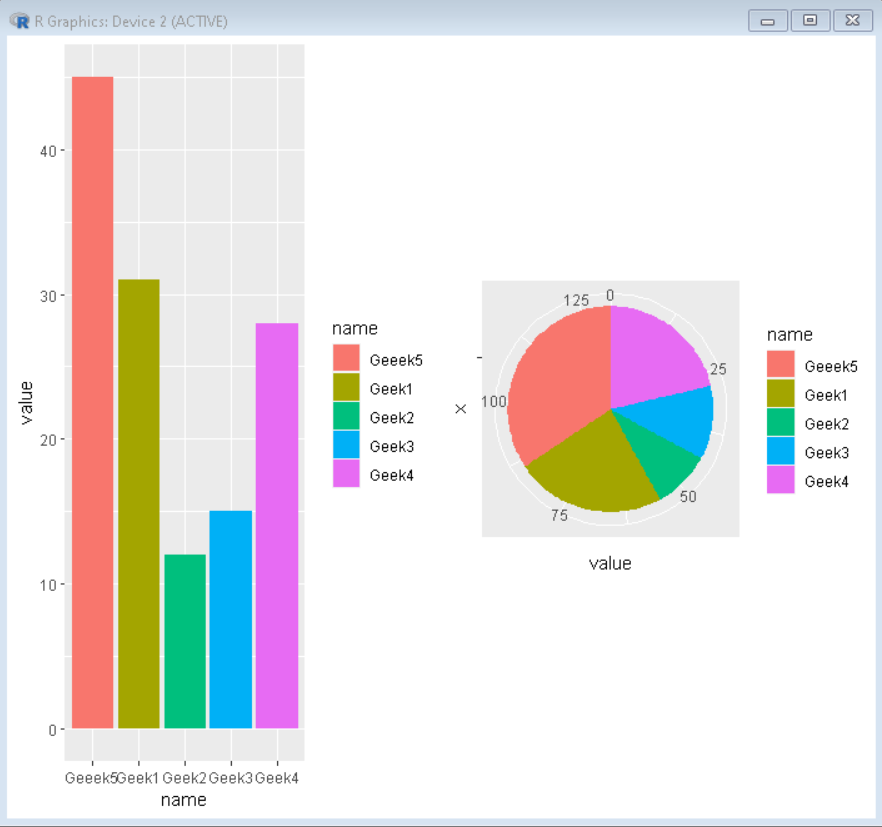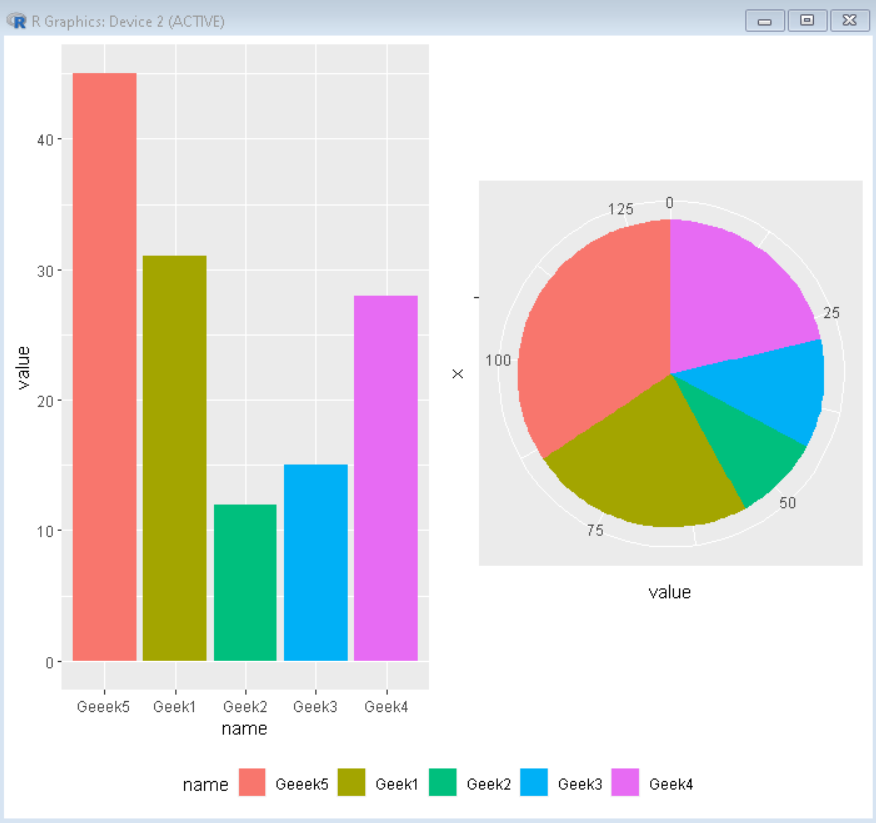# Shared legend for a cowplot grid in R

• Last Updated : 24 Oct, 2021

In this article, we will discuss how to create a cowplot grid with the shared legend in the R Programming language.

To do this first we will make a basic cowplot grid with two plots having their own legends separately. To do so using the cowplot grid package in the R Language we use the plot_grid() function and pass all the plots that we need in the grid as parameters.

Syntax: plot_grid(plot1,plot2,label=<label-vector>, ncol, nrow)

Example:

A basic cowplot grid with two plots having separate legends.

## R

 `# Create sample data                            ` `sample_data <- ``data.frame``( ` `  ``name = ``c``(``"Geek1"``,``"Geek2"``,``"Geek3"``, ` `           ``"Geek4"``,``"Geeek5"``) , ` `  ``value=``c``(31,12,15,28,45)) ` ` `  `# Load ggplot2 and cowplot ` `library``(``"ggplot2"``)  ` `library``(``"cowplot"``) ` ` `  `# Create both plot and store in variable ` `plot1<-``ggplot``(sample_data, ``aes``(x=name, y=value, fill=name)) + ` `        ``geom_bar``(stat = ``"identity"``) ` ` `  `plot2<-``ggplot``(sample_data, ``aes``(x=``""``, y=value, fill=name))+ ` `        ``geom_bar``(width = 1, stat = ``"identity"``) + ` `        ``coord_polar``(``"y"``, start=0) ` ` `  `# plot_grid() function adds both plots in a cowplot grid ` `plot_grid``(plot1, plot2)`

Output:## Shared Legend cowplot grid

To create a cowplot grid with shared legend there is no built-in method but the functionality can be achieved by following these steps:

Step 1: Create plots to be put in the grid without legend using:

`plot + theme(legend.position = "none")`

Step 2: Now combine both plots using the plot_grid() function and store that in a variable:

`combined_plot<-plot_grid(plot1, plot2, ...... ncol)`

Step 3: Now we extract the legend from one of the above plots to put it in the combined plot using:

`legend <- get_legend( plot1 )`

Step 4: Finally combine the combined plot with the derived legend using the plot_grid function to get the desired look for the shared legend plot.

`plot_grid(combined_plot, legend, ncol)`

Example:

Here, is an implementation of the above approach to get a cowplot grid with shared legend.

## R

 `# Create sample data                            ` `sample_data <- ``data.frame``( ` `  ``name = ``c``(``"Geek1"``,``"Geek2"``,``"Geek3"``, ` `           ``"Geek4"``,``"Geeek5"``) , ` `  ``value=``c``(31,12,15,28,45)) ` ` `  `# Load ggplot2 and cowplot ` `library``(``"ggplot2"``)  ` `library``(``"cowplot"``) ` ` `  `# Create both plot without legend and store in variable ` `plot1<-``ggplot``(sample_data, ``aes``(x=name, y=value, fill=name)) + ` `        ``geom_bar``(stat = ``"identity"``)+ ` `        ``theme``(legend.position = ``"none"``) ` ` `  `plot2<-``ggplot``(sample_data, ``aes``(x=``""``, y=value, fill=name))+ ` `        ``geom_bar``(width = 1, stat = ``"identity"``) + ` `        ``coord_polar``(``"y"``, start=0)+ ` `        ``theme``(legend.position = ``"none"``) ` ` `  `# combine both plot using plot_grid() ` `combined_plot<-``plot_grid``(plot1, plot2,ncol=2) ` ` `  `# extract legend from plot1 ` `legend <- ``get_legend``( ` `  ``plot1 + ` `    ``guides``(color = ``guide_legend``(nrow = 1)) + ` `    ``theme``(legend.position = ``"bottom"``) ` `) ` ` `  `# Combine combined plot and legend using plot_grid() ` `plot_grid``(combined_plot, legend,ncol=1,rel_heights = ``c``(1, .1))`

Output:My Personal Notes arrow_drop_up
Recommended Articles
Page :